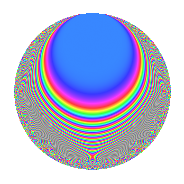# Properties

 Label 41.2.gLevel 41 Weight 2 Character orbit g Rep. character $$\chi_{41}(2,\cdot)$$ Character field $$\Q(\zeta_{20})$$ Dimension 24 Newform subspaces 1 Sturm bound 7 Trace bound 0

# Related objects

## Defining parameters

 Level: $$N$$ = $$41$$ Weight: $$k$$ = $$2$$ Character orbit: $$[\chi]$$ = 41.g (of order $$20$$ and degree $$8$$) Character conductor: $$\operatorname{cond}(\chi)$$ = $$41$$ Character field: $$\Q(\zeta_{20})$$ Newform subspaces: $$1$$ Sturm bound: $$7$$ Trace bound: $$0$$

## Dimensions

The following table gives the dimensions of various subspaces of $$M_{2}(41, [\chi])$$.

Total New Old
Modular forms 40 40 0
Cusp forms 24 24 0
Eisenstein series 16 16 0

## Trace form

 $$24q - 10q^{2} - 6q^{3} - 10q^{5} - 2q^{6} - 8q^{7} - 10q^{8} + O(q^{10})$$ $$24q - 10q^{2} - 6q^{3} - 10q^{5} - 2q^{6} - 8q^{7} - 10q^{8} + 6q^{10} - 16q^{11} + 2q^{12} + 14q^{14} + 8q^{15} - 20q^{16} + 8q^{17} + 16q^{19} + 20q^{20} - 10q^{21} + 6q^{22} + 12q^{23} + 68q^{24} - 8q^{25} - 28q^{26} - 6q^{27} + 18q^{28} + 40q^{29} - 36q^{30} - 12q^{31} + 10q^{33} - 16q^{34} - 36q^{35} - 40q^{36} + 46q^{38} - 50q^{39} - 44q^{40} - 4q^{41} - 40q^{42} - 48q^{44} + 16q^{45} + 70q^{46} - 12q^{47} - 50q^{48} - 30q^{49} - 24q^{51} + 20q^{52} - 26q^{53} + 68q^{54} + 20q^{55} + 106q^{56} + 10q^{57} - 20q^{58} + 6q^{59} + 76q^{60} + 30q^{61} - 10q^{62} + 92q^{63} + 70q^{64} + 68q^{65} + 34q^{66} - 22q^{67} - 20q^{68} - 38q^{69} - 20q^{70} + 4q^{71} - 74q^{72} + 10q^{74} + 4q^{75} - 128q^{76} - 20q^{77} - 10q^{78} - 2q^{79} - 70q^{80} + 28q^{81} - 90q^{82} + 80q^{83} - 30q^{84} - 56q^{85} - 46q^{86} - 10q^{87} + 10q^{88} - 72q^{89} - 70q^{90} - 6q^{93} - 18q^{94} - 40q^{95} + 66q^{96} - 22q^{97} + 6q^{98} + 14q^{99} + O(q^{100})$$

## Decomposition of $$S_{2}^{\mathrm{new}}(41, [\chi])$$ into newform subspaces

Label Dim. $$A$$ Field CM Traces $q$-expansion
$$a_2$$ $$a_3$$ $$a_5$$ $$a_7$$
41.2.g.a $$24$$ $$0.327$$ None $$-10$$ $$-6$$ $$-10$$ $$-8$$

## Hecke Characteristic Polynomials

There are no characteristic polynomials of Hecke operators in the database